# Fair-by-design matching

## Abstract

Matching algorithms are used routinely to match donors to recipients for solid organs transplantation, for the assignment of medical residents to hospitals, record linkage in databases, scheduling jobs on machines, network switching, online advertising, and image recognition, among others. Although many optimal solutions may exist to a given matching problem, when the elements that shall or not be included in a solution correspond to individuals, it becomes of paramount importance that the solution is selected fairly. In this paper we study individual fairness in matching problems. Given that many maximum matchings may exist, each one satisfying a different set of individuals, the only way to guarantee fairness is through randomization. Hence we introduce the distributional maxmin fairness framework which provides, for any given input instance, the strongest guarantee possible simultaneously for all individuals in terms of satisfaction probability (the probability of being matched in the solution). Specifically, a probability distribution over feasible solutions is maxmin-fair if it is not possible to improve the satisfaction probability of any individual without decreasing it for some other individual which is no better off. Our main contribution is a polynomial-time algorithm building on techniques from minimum cuts, and edge-coloring algorithms for regular bipartite graphs, and transversal theory. In the special case of bipartite matching, our algorithm runs in $$O((|V|^2 + |E| |V|^{2/3})\cdot (\log |V|)^2)$$ expected time. An experimental evaluation of our fair-matching algorithm shows its ability to scale to graphs with tens of millions of vertices and hundreds of millions of edges, taking only a few minutes on a simple architecture. To the best of our knowledge, this yields the first large-scale implementation of the egalitarian mechanism of Bogomolnaia and Moulin (Econometrica 72(1):257–279, 2004). Our analysis confirms that our method provides stronger satisfaction probability guarantees than non-trivial baselines.

This is a preview of subscription content, access via your institution.

1. 1.

Each of these distributions can be represented by a list of pairs (probabilitymatching), with the probabilities being non-negative and summing up to 1. For our second algorithm a simpler representation is possible: the distribution of matchings $$D_i$$ within each block (but not for the whole graph) is uniform over some small multiset of matchings.

## References

1. Big data: Seizing opportunities, preserving values. Technical report, US Executive Office of the President (2014). https://obamawhitehouse.archives.gov/sites/default/files/docs/big_data_privacy_report_may_1_2014.pdf. Accessed 21 Jan 2019

2. Big data: A report on algorithmic systems, opportunity, and civil rights. Technical report, US Executive Office of the President (2016). https://obamawhitehouse.archives.gov/blog/2016/05/04/big-risks-big-opportunities-intersection-big-data-and-civil-rights. Accessed 21 Jan 2019

3. Bansal N, Sviridenko M (2006) The Santa Claus problem. In: Proceedings of the thirty-eighth annual ACM symposium on theory of computing, STOC ’06. ACM, New York, NY, USA, pp 31–40. https://doi.org/10.1145/1132516.1132522

4. Berge C (1957) Two theorems in graph theory. PNAS 43(9):842–844

5. Bertsekas DP, Gallager RG, Humblet P (1992) Data networks, vol 2. Prentice-Hall International, Upper Saddle River

6. Bertsimas D, Farias VF, Trichakis N (2011) The price of fairness. Oper Res 59(1):17–31

7. Birkhoff G (1946) Tres observaciones sobre el álgebra lineal. Univ Nac Tucumán Rev Ser A 5:147–151

8. Bogomolnaia A, Moulin H (2001) A new solution to the random assignment problem. J Econ Theory 100(2):295–328

9. Bogomolnaia A, Moulin H (2004) Random matching under dichotomous preferences. Econometrica 72(1):257–279

10. Brams SJ, Jones MA, Klamler C et al (2006) Better ways to cut a cake. Notices AMS 53(11):1314–1321

11. Brânzei S, Gkatzelis V, Mehta R (2017) Nash social welfare approximation for strategic agents. In: EC. ACM, pp 611–628

12. Brown JR (1979) The sharing problem. Oper Res 27(2):324–340

13. Caragiannis I, Kurokawa D, Moulin H, Procaccia AD, Shah N, Wang J (2016) The unreasonable fairness of maximum Nash welfare. In: EC

14. Charikar M (2000) Greedy approximation algorithms for finding dense components in a graph. In: APPROX, pp 84–95

15. Cheng CT (2010) Understanding the generalized median stable matchings. Algorithmica 58(1):34–51

16. Cherkassky BV, Goldberg AV (1997) On implementing the push-relabel method for the maximum flow problem. Algorithmica 19(4):390–410

17. Cherkassky BV, Goldberg AV (2004) HIPR 3.5. Technical report, IG systems, Inc. http://www.avglab.com/andrew/soft.html. Accessed 21 Jan 2019

18. Cole R, Ost K, Schirra S (2001) Edge-coloring bipartite multigraphs in O (E log D) time. Combinatorica 21(1):5–12

19. Coluccia A, D’Alconzo A, Ricciato F (2012) On the optimality of max–min fairness in resource allocation. Ann Telecommun 67(1–2):15–26

20. Corbett-Davies S, Pierson E, Feller A, Goel S, Huq A (2017) Algorithmic decision making and the cost of fairness. In: KDD

21. Dulmage AL, Mendelsohn NS (1958) Coverings of bipartite graphs. Can JRL Math 10(4):516–534

22. Dwork C (2017) What’s fair? In: KDD [Keynote]

23. Dwork C, Hardt M, Pitassi T, Reingold O, Zemel R (2012) Fairness through awareness. In: Proceedings of the 3rd conference on innovations in theoretical computer science. ACM, pp 214–226

24. Edmonds J (1965) Paths, trees, and flowers. Can JRL Math 17(3):449–467

25. Edmonds J (1971) Matroids and the greedy algorithm. Math Prog 1(1):127–136

26. Edmonds J, Fulkerson D (1965) Transversals and matroid partition. J Res Nat Bur Stand 69B:147–153

27. Edmonds J, Pruhs K (2006) Cake cutting really is not a piece of cake. In: SODA. Society for Industrial and Applied Mathematics, pp 271–278

28. Feldman M, Friedler SA, Moeller J, Scheidegger C, Venkatasubramanian S (2015) Certifying and removing disparate impact. In: KDD

29. Gale D, Shapley LS (1962) College admissions and the stability of marriage. Am Math Mon 69(1):9–15

30. Gallo G, Grigoriadis MD, Tarjan RE (1989) A fast parametric maximum flow algorithm and applications. SIAM J Comput 18(1):30–55

31. Goel A, Kapralov M, Khanna S (2013) Perfect matchings in $$O(n\,\log n)$$ time in regular bipartite graphs. SIAM J Comput 42(3):1392–1404

32. Goldberg AV, Rao S (1998) Beyond the flow decomposition barrier. J ACM 45(5):783–797

33. Goldberg AV, Tarjan RE (1988) A new approach to the maximum-flow problem. J ACM (JACM) 35(4):921–940

34. Hall P (1935) On representatives of subsets. JRL Lond Math Soc 1(1):26–30

35. Hardy GH, Littlewood JE, Pólya G (1952) Inequalities. CUP

36. Heidari H, Ferrari C, Gummadi KP, Krause A (2018) Fairness behind a veil of ignorance: a welfare analysis for automated decision making. In: Neural and information processing systems (NeurIPS)

37. Heidari H, Loi M, Gummadi KP, Krause A (2019) A moral framework for understanding of fair ml through economic models of equality of opportunity. In: ACM conference on fairness, accountability, and transparency (ACM FAT*)

38. Ichimori T, Ishii H, Nishida T (1982) Optimal sharing. Math Program 23(1):341–348

39. Joseph M, Kearns M, Morgenstern JH, Roth A (2016) Fairness in learning: classic and contextual bandits. In: Advances in neural information processing systems, pp 325–333

40. Kamada Y, Kojima F (2015) Efficient matching under distributional constraints: theory and applications. Am Econ Rev 105(1):67–99

41. Kamiran F, Calders T (2011) Data preprocessing techniques for classification without discrimination. Knowl Inf Syst 33(1):1–33

42. Katoh N, Ibaraki T, Mine H (1985) An algorithm for the equipollent resource allocation problem. Math Oper Res 10(1):44–53

43. Kearns M (2017) Fair algorithms for machine learning. In: EC

44. Klamler C (2010) Fair division. In: Kilgour DM, Eden C (eds) Handbook of group decision and negotiation. Springer, Berlin, pp 183–202

45. Lawler EL (1976) Combinatorial optimization: networks and matroids. Courier Corporation, North Chelmsford

46. Lovász L, Plummer M (2009) Matching theory. AMS Chelsea Publishing Series, North-Holland

47. McElfresh DC, Dickerson JP (2018) Balancing lexicographic fairness and a utilitarian objective with application to kidney exchange. In: Thirty-second AAAI conference on artificial intelligence

48. Megiddo N (1977) A good algorithm for lexicographically optimal flows in multi-terminal networks. Bull Am Math Soc 83(3):407–409

49. Pedreschi D, Ruggieri S, Turini F (2008) Discrimination-aware data mining. In: KDD

50. Picard JC, Queyranne M (1980) On the structure of all minimum cuts in a network and applications. In: Rayward-Smith VJ (ed) Combinatorial optimization II. Mathematical programming studies, vol 13. Springer, Berlin, Heidelberg

51. Rawls J (1971) A theory of justice. Harvard University Press, Cambridge

52. Roth AE, Sönmez T, Ünver MU (2005) Pairwise kidney exchange. J Econ Theory 125(2):151–188

53. Teo CP, Sethuraman J (1998) The geometry of fractional stable matchings and its applications. Math Oper Res 23(4):874–891

54. Triesch E (1992) A short proof that matching matroids are transversal. Appli Math Lett 5(2):19–20

55. Zliobaite I, Kamiran F, Calders T (2011) Handling conditional discrimination. In: ICDM

## Author information

Authors

### Corresponding author

Correspondence to David García-Soriano.

### Publisher's Note

Springer Nature remains neutral with regard to jurisdictional claims in published maps and institutional affiliations.

Responsible editor: Karsten Borgwardt, Po-Ling Loh, Evimaria Terzi, Antti Ukkonen.

## Appendices

### Appendix A: Proofs for Sect. 3: fairness and social inequality

Preliminaries In order to prove Theorem 2, we need to recall some additonal facts about matroids [refer to Lawler (1976) for details]. The rank function $$\rho :2^L \rightarrow \mathbb {N}$$ of a matroid is monotone submodular, meaning that for all $$S, T\subseteq L$$, it holds that $$0 \le \rho (S \cup T) - \rho (S) \le \rho (T) - \rho (S \cap T).$$ The dual matroid of M is the matroid with ground set L given by $$M^* = \{ L {\setminus } S \mid S \in M \}$$; clearly the dual of $$M^*$$ is M itself. The rank function of $$M^*$$ is given by $$\rho ^*(S) = |S| - (\rho (L) - \rho (L{\setminus } S))$$. The contraction of M to the set $$L {\setminus } S$$ is the matroid M/S with ground set $$L {\setminus } S$$ and rank function $$\rho _{M/S}(X) = \rho (S \cup X) - \rho (S).$$ The restriction of M to the set S is the matroid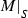with ground set S and independent sets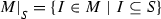.

### Proof of Lemma 1

Assume that the set

\begin{aligned} A = \{u \in {{\mathcal {U}}}\mid {F}[{u}] \ne {D}[{u}]\} \end{aligned}

is non-empty. Let

\begin{aligned} u = {{\,\mathrm{arg\,min}\,}}\{\, \min ({F}[{u}], {D}[{u}]) \mid u\in A\, \}, \end{aligned}

where ties are broken arbitrarily. Then $${D}[{u}] \ne {F}[{u}]$$; suppose that $${D}[{u}] > {F}[{u}]$$. Then for any $$v \in A$$, our choice of u implies that $${D}[{v}] \ge \min ({D}[{v}], {F}[{v}]) \ge \min ({D}[{u}], {F}[{u}]) = {F}[{u}]$$; and for any $$v\notin A$$, we have $${D}[{v}] = {F}[{v}]$$ by definition. In either case one of the inequalities required by condition (1) fails, so F is not maxmin-fair. Put differently, we have shown the following implication:

\begin{aligned} F \text { is maxmin-fair } \implies {D}[{u}] < {F}[{u}]. \end{aligned}

Similarly,

\begin{aligned} D \text { is maxmin-fair } \implies {F}[{u}] < {D}[{u}]. \end{aligned}

But then F and D cannot both be maxmin-fair. The only way out of this contradiction is to conclude that A is empty.

### Proof of Theorem 1

$$\implies$$ Let F be maxmin-fair and consider any other distribution D. We need to show that $${F\uparrow \,} \succeq {D\uparrow \,}$$ (that is, $${F\uparrow \,}$$ is lexicographically largest). Define

\begin{aligned} A = \{u \in {{\mathcal {U}}}\mid {F}[{u}] \ne {D}[{u}]\}. \end{aligned}

If A is empty, the claim is trivial; otherwise let

\begin{aligned} u = {{\,\mathrm{arg\,min}\,}}\{ {F}[{u}] \mid u\in A \} \; \text { and } \; B = \{v \in {{\mathcal {U}}}\mid {F}[{v}] < {F}[{u}]\}. \end{aligned}

Note that $$u \in A\subseteq {\overline{B}}$$ by our choice of u. If $${D}[{u}] > {F}[{u}]$$, from the maxmin-fairness of F we infer the existence of $$v\in A\subseteq {\overline{B}}$$ such that $${D}[{v}] < {F}[{u}]$$. This also holds if $${D}[{u}] < {F}[{u}]$$ (then we can take $$v = u$$). In any case we have

\begin{aligned} \min \{ {D}[{v}] \mid v \notin B \}< {F}[{u}] = \min \{ {F}[{v}] \mid v \notin B \} \\ \text { and } \qquad {D}[{v}] = {F}[{v}] < {F}[{u}] \; \forall v \in B. \end{aligned}

It is readily verified that this implies $${F\uparrow \,} \succ {D\uparrow \,}$$.

$$\Longleftarrow$$ Let F be a distribution which is not maxmin-fair. We show that F is not lexicographically largest either. Since (1) does not hold for F, there exists another distribution D and a user $$u \in {{\mathcal {U}}}$$ such that

\begin{aligned} {D}[{u}]> {F}[{u}] \text { and } ({D}[{v}] < {F}[{v}] \implies {F}[{v}] > {F}[{u}]) \; \forall v. \end{aligned}
(6)

For any $$\varepsilon \in (0, 1)$$, let $$X_\varepsilon$$ denote the distribution picking F with probability $$1-\varepsilon$$ and D with probability $$\varepsilon$$, so that

\begin{aligned} {X_\varepsilon }[{v}] = {F}[{v}] + \varepsilon ({D}[{v}] - {F}[{v}])\quad \forall v. \end{aligned}

Choose $$\varepsilon > 0$$ small enough so as to guarantee that

\begin{aligned} ({F}[{v}]< {F}[{u}] \implies {X_{\varepsilon }}[{v}] < {X_{\varepsilon }}[{u}]) \quad \forall v \end{aligned}
(7)

and

\begin{aligned} ({F}[{v}]> {F}[{u}] \implies {X_{\varepsilon }}[{v}] > {X_{\varepsilon }}[{u}]) \quad \forall v. \end{aligned}
(8)

For instance, any

\begin{aligned} \varepsilon < \min \left\{ \frac{|{F}[{u}] - {F}[{v}]|}{|{D}[{v}] - {F}[{v}]| + |{D}[{u}] - {F}[{u}]|} \Big \vert {F}[{v}] \ne {F}[{u}] \right\} \end{aligned}

will do. We have, by (6),

\begin{aligned} ({F}[{v}] \le {F}[{u}] \implies {D}[{v}] \ge {F}[{v}] \implies {X_{\varepsilon }}[{v}] \ge {F}[{v}]) \quad \forall v \end{aligned}
(9)
\begin{aligned} \text {and} \qquad {D}[{u}] > {F}[{u}]. \end{aligned}
(10)

But (7), (8), (9) and (10) say that $${X_\varepsilon \uparrow \,}$$ is strictly larger than $${F\uparrow \,}$$ in lexicographical order, as we wished to show.

The following two analogues of Lemma 1 and Theorem 1 are also needed for the proof of Theorem 2:

### Lemma 7

If F and D are both minmax-Pareto, then $${F}[{u}] = {D}[{u}]$$ for all $$u \in {{\mathcal {U}}}$$.

### Proof

Assume that the set

\begin{aligned} A = \{u \in {{\mathcal {U}}}\mid {F}[{u}] \ne {D}[{u}]\} \end{aligned}

is non-empty. Let

\begin{aligned} u = {{\,\mathrm{arg\,max}\,}}\{\, \max ({F}[{u}], {D}[{u}]) \mid u\in A\, \}, \end{aligned}

where ties are broken arbitrarily. Then $${D}[{u}] \ne {F}[{u}]$$; suppose that $${D}[{u}] < {F}[{u}]$$. Then for any $$v \in A$$, our choice of u implies that $${D}[{v}] \le \max ({D}[{v}], {F}[{v}]) \le \max ({D}[{u}], {F}[{u}]) = {F}[{u}]$$; and for any $$v\notin A$$, we have $${D}[{v}] = {F}[{v}]$$ by definition. In either case one of the inequalities required by the definition of minmax-Pareto efficiency fails. Put differently, we have shown the following implication:

\begin{aligned} F \text { is minmax-Pareto } \implies {D}[{u}] > {F}[{u}]. \end{aligned}

Similarly,

\begin{aligned} D \text { is minmax-Pareto } \implies {F}[{u}] > {D}[{u}]. \end{aligned}

But then F and D cannot both be minmax-Pareto. The only way out of this contradiction is to conclude that A is empty. $$\square$$

### Theorem 14

For matroid problems, a distribution F is minmax-Pareto if and only if F is Pareto-efficient and $${F\downarrow \,} \preceq {D\downarrow \,}$$ for all Pareto-efficient distributions D.

### Proof

First observe that, for matroids, a distribution is Pareto-efficient if and only if it is supported over bases. For any distribution D of bases over a matroid M with ground set L, consider the distribution $$D^*$$ of $$(L {\setminus } X \mid X \sim D)$$ of bases over the dual matroid $$M^*$$. Then we have $${D}[{u}] + {D^*}[{u}] = 1$$ for all $$u \in L$$, so clearly F is minmax-Pareto if and only if $$F^*$$ is maxmin-fair, which (by Theorem 1) occurs if and only if $$F^*$$ is lexicographically largest for $$M^*$$, which in turn is equivalent to F being lexicographically smallest among distributions of bases of M, as we wished to show. $$\square$$

Our next result asserts that the only obstruction to achieving high satisfaction probability for every user is the existence of a set of users with small rank-to-size ratio. Finding these obstruction sets will enable us to devise a divide and conquer strategy to obtain fair distributions. For instance, in Example 1 the obstruction set is given by the set of users $$\{a_1, a_2, a_3\}$$, which force the maximum satisfaction probability to be no larger than $$\frac{2}{3}$$.

### Theorem 15

Let M be a matroid with ground set L and rank function $$\rho :2^L \rightarrow \mathbb {N}$$. The minimum satisfaction probability in a minmax-fair distribution over M is

\begin{aligned} \pi (M) = \min \left\{ \frac{\rho (X)}{|X|} \mid \emptyset \ne X \subseteq L \right\} . \end{aligned}

### Proof

Any maxmin-fair distribution is supported on the collection $$\mathcal {B}$$ of bases of M, since extending an independent set to a base containing it never decreases any satisfaction probability. Optimizing the smallest satisfaction probability $$\lambda _1$$ amounts to finding a suitable distribution over $$\mathcal {B}$$; let us denote the corresponding probabilities by $$\{p_B\}_{B \in \mathcal {B}}$$. Since the probability of $$v \in {{\mathcal {U}}}$$ being included is $$\sum _{v \in B} p_B$$, maximizing the minimum such probability is modeled by Program (11) below. It may be written as a linear program by introducing an additional variable $$\lambda$$ to be maximized, and introducing the constraints $$\sum _{B \ni v} p_B \ge \lambda$$. Its dual is equivalent to (12).

\begin{aligned} \begin{array}{rrclcl} \displaystyle \max &{} \displaystyle \min _{v\in {{\mathcal {U}}}} \sum _{B \ni v} p_B \\ \\ \text {s.t.} &{} \displaystyle \sum _{B \in \mathcal {B}} p_B &{} = &{} 1 &{} \\ &{} p_B &{}\ge &{} 0 \\ \end{array} \end{aligned}
(11)
\begin{aligned} \begin{array}{rrclcl} \displaystyle \min &{} \displaystyle \max _{B\in \mathcal {B}} \displaystyle \sum _{v \in B} z_v \\ \\ \text {s.t.} &{} \displaystyle \sum _{v \in {{\mathcal {U}}}} z_v &{} = &{} 1 &{} \\ &{} z_v &{}\ge &{} 0. \\ \end{array} \end{aligned}
(12)

Observe that $$\max _{B\in \mathcal {B}} \sum _{v\in \mathcal {B}} z_v$$ is the value of a maximum-weight base of M, with weights given by $$\{z_v\}_{v \in {{\mathcal {U}}}}$$. Thus LP (12) encodes the task of finding an assignment of weights to elements of $${{\mathcal {U}}}$$ minimizing the maximum weight of a base. We will turn this min–max problem into a pure minimization problem.

Edmonds (1971) showed that for any fixed assignment of non-negative weights to the elements of M, a maximum-weight base may be found via the greedy algorithm that examines each element in order of decreasing weight and adds it to the current set if its addition does not violate independence. Let $$\varPi$$ denote the set of permutations of $${{\mathcal {U}}}= \{1, 2, \ldots , n\}$$. Write

\begin{aligned} \varDelta = \left\{ z \in \mathbb {R}^{{\mathcal {U}}}\big \vert \sum _{v \in {{\mathcal {U}}}} z_v = 1, \quad z_v \ge 0 \; \forall v \in {{\mathcal {U}}}\right\} \end{aligned}

for the probability simplex on $${{\mathcal {U}}}$$ and let $$G(\pi ) = \{ z \in \varDelta \mid z_{\pi (1)} \ge z_{\pi (2)} \ge \cdots \ge z_{\pi (n)} \}$$ denote the elements of $$\varDelta$$ which become sorted after applying permutation $$\pi \in \varPi$$.

Note that if $$z, z' \in G(\pi )$$, then the two bases obtained via the greedy algorithm with vertex weights $$\{z_v\}$$ and $$\{z'_v\}$$ are the same. For each $$\pi \in \varPi$$, let $$B(\pi )$$ denote the base obtained via the greedy algorithm; Edmond’s result may then be written as

\begin{aligned} \max _{B \in \mathcal {B}} \sum _{v \in B} z_v = \sum _{v \in B(\pi )} z_v \quad \text { if } z \in G(\pi ). \end{aligned}

By LP (12), the fairness parameter $$\pi (M)$$ is

\begin{aligned} \min _{z \in \varDelta }\; \max _{B \in \mathcal {B}} \sum _{v \in B} z_v = \min _{\pi \in \varPi }\, \min _{z \in G(\pi )}\, \max _{B \in \mathcal {B}} \sum _{v \in B} z_v = \min _{\pi \in \varPi }\, \min _{z \in G(\pi )}\, \sum _{v \in B(\pi )} z_v. \end{aligned}
(13)

We claim that, for each $$\pi \in \varPi$$ and each non-empty $$X \subseteq {{\mathcal {U}}}$$,

\begin{aligned} \min _{z \in G(\pi )} \sum _{v \in X} z_v = \min _{i \in [n]} \frac{|X \cap \pi ([i])|}{i}, \end{aligned}
(14)

where $$\pi ([i]) = \{ \pi (1), \ldots , \pi (i) \}$$.

This means that, if we are given advice on the permutation $$\pi$$ which sorts an optimal solution z to LP (12), then we can find another solution $${\hat{z}}$$ with the same value and whose non-zero weights are evenly distributed among the top t elements of z in sorted order, for some $$t \in [n]$$. For some optimal t, each of the t non-zero values of $${\hat{z}}_i$$ is either 0 or 1/t. To see this assuming 14, notice that we can construct such $${\hat{z}}$$ by setting $${\hat{z}}_{\pi (i)} = \frac{1}{t}$$ for $$1 \le i \le t$$ and $${\hat{z}}_j = 0$$ for $$j \notin \pi ([t])$$.

To see why (14) holds, define $$d_{n} = z_{\pi (n)}$$ and $$d_i = z_{\pi (i)} - z_{\pi (i+1)} \ge 0$$ for $$0< i < n$$. Then $$z_{\pi (i)} = \sum _{j \ge i} d_i,$$ hence

\begin{aligned} \sum _{v \in X} z_v = \sum _{i\in [n]} \left( \mathbb {1}[{\pi (i) \in X}] \cdot \sum _{j \ge i} d_i \right) = \sum _{j\in [n]} d_j \cdot |X \cap \pi ([j])|. \end{aligned}

The conditions $$\sum _{v \in {{\mathcal {U}}}} z_v = 1$$ and $$z \in G(\pi )$$ then become $$\sum _i i \cdot d_i = 1$$ and $$d_i \ge 0$$. Therefore

\begin{aligned} \min _{z \in G(\pi )} \sum _{v \in X} z_v = \min \left\{ \sum _{i\in [n]} d_i \cdot |X \cap \pi ([i])| \;\Big \vert \; \sum _{i\in [n]} i \cdot d_i = 1, d_i \ge 0 \right\} . \end{aligned}

The quantity in the right-hand side equals the smallest ratio (among all i) between the coefficient of $$d_i$$ in the objective function ($$|X \cap \pi ([i])|$$) and in the only equality constraint, proving (14). From (13) and (14) the theorem follows, because the greedy algorithm satisfies $$|B(\pi ) \cap \pi ([i])| = \rho (\pi [i])$$ for all i, so if $$\displaystyle S^*= {{\,\mathrm{arg\,min}\,}}_{S\subseteq {{\mathcal {U}}}}\frac{ \rho (S) }{ |S|}$$, then for any $$\pi \in \varPi$$ we have

\begin{aligned} \min _{z \in G(\pi )} \sum _{v \in B(\pi )} z_v =\min _{i \in [n]} \frac{|B(\pi ) \cap \pi ([i])|}{i} = \min _{i \in [n]} \frac{\rho (\pi ([i]))}{|\pi ([i])|} \ge \frac{\rho (S^*)}{|S^*|}, \end{aligned}

and equality holds for any permutation where the elements of $$S^*$$ precede those of $${{\mathcal {U}}}{\setminus } S^*$$. $$\square$$

### Theorem 16

Let M be a matroid with ground set L and rank function $$\rho :2^L \rightarrow \mathbb {N}$$. The maximum satisfaction probability in a minmax-Pareto distribution over M is

\begin{aligned} \varPi (M) = \max \left\{ \frac{\rho (L) - \rho (X)}{|L {\setminus } X|} \mid {\emptyset \subseteq X \subsetneq L} \right\} . \end{aligned}

### Proof

Apply Theorem 15 to the dual matroid of M. $$\square$$

An extension of Theorem 15 allows us to compute the satisfaction probability of every element of L.

### Lemma 8

Define a sequence of sets $$B_1, B_2, \ldots , B_k$$ iteratively by:

\begin{aligned}&B_i \text { is a maximal set } X \subseteq L{\setminus } S_{i-1} \text { minimizing } \frac{ \rho (X \cup S_{i-1}) - \rho (S_{i-1}) }{|X|} ,\nonumber \\&\quad \text { where } S_i = \bigcup _{j=1}^i B_j. \end{aligned}
(15)

We stop when $$S_i = L$$ (which will eventually occur as the sequence $$\{S_i\}$$ is strictly increasing). Then for every $$i, u \in B_i$$, the satisfaction probability of u in a maxmin-fair distribution F is $$\lambda _i = \frac{ \rho (B_i) }{ |B_i| }$$.

(Maximality of each $$B_i$$ is not required for the conclusion to hold, but its inclusion guarantees uniqueness of the sets thus defined, owing to the submodularity of $$\rho$$.)

### Proof

We reason by induction on the number k of sets. First, observe that Theorem 15 implies $$F[u] \ge \lambda _1$$ for all $$u \in L$$. As the expected number of satisfied elements within $$B_1$$, which obviously cannot exceed $$\rho (B_1) = \lambda _1 |B_1|$$, is equal to

\begin{aligned} {{\,\mathrm{\mathbb {E}}\,}}_{A \sim F} [|A \cap B_1|] = \sum _{u\in B_1} F[u] \ge \lambda _1 |B_1| \end{aligned}
(16)

by linearity of expectation, the equality $$F[u] = \lambda _1$$ must hold for all $$u \in B_1$$. If $$k = 1$$, this shows the result.

If $$k > 1$$, let $$D_1$$ be a maxmin-fair distribution for the restriction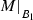of M to $$B_1$$ and let $$D_2$$ be a maxmin-fair distribution for the contraction $$M/(L{\setminus } B_1)$$ of M to the remaining elements $$L {\setminus } B_1$$. Since restriction does not change the rank function within $$B_1$$, $$D_1$$ satisfies $$D_1[u] = F[u] = \lambda _1$$ for all $$u \in B_1$$. The rank function of the contraction $$M/(L{\setminus } B_1)$$ is $$\rho _{M/B_1}(X) = \rho (X \cup B_1) - \rho (B_1)$$, so by applying rule (15) iteratively we obtain the same sequence of sets $$B_2, \ldots , B_k$$ (excluding $$B_1$$), and by the induction hypothesis $$D_2$$ satisfies $$D_2[u] = \lambda _i$$ for all $$i\ge 2, u \in B_i$$. It remains to be shown that $$D_2[u] = F[u]$$ for all $$u \notin B_1$$.

Denote by $$[D_1 \cup D_2]$$ the distribution of $$(A \cup B \mid A \sim D_1, B \sim D_2)$$. This is a distribution over independent sets of M by the following property of matroid contractions [see Lawler (1976)]: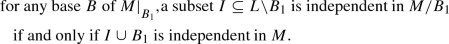(17)

On the other hand, for any set A in the support of a maxmin-fair distribution F, the set $$A \cap B_1$$ must be a base of(or else Eq. (16) would fail). Let $$F_2$$ denote the distribution $$(A {\setminus } B_1 \mid A \sim F)$$; by (17), $$F_2$$ is a distribution over elements of the contraction $$M/B_1$$.

To complete the proof, observe that $$F\uparrow \succeq [D_1 \cup D_2]\uparrow$$ by Theorem 1 because F is maxmin-fair. As $${F}[{u}] = {D_1}[{u}] < {F}[{v}], {D}[{v}]$$ for $$u \in B_1, v \in L{\setminus } B_1$$, the fact that $$F\uparrow \succeq [D_1 \cup D_2]\uparrow$$ implies $$F_2\uparrow \succeq D_2\uparrow$$, and the maxmin-fairness of $$D_2$$ allows us to deduce that $$F_2\uparrow = D_2\uparrow$$. Hence, by Lemma 1, for all $$v \notin B_1$$ we have $${F}[{v}] = {F_2}[{v}] = {D_2}[{v}]$$.

$$\square$$

Similarly, we have the following for minmax-fairness.

### Lemma 9

Define a sequence of sets $$B'_1, \ldots , B'_{k'}$$ iteratively by:

\begin{aligned}&B'_i \text { is a maximal set } X\subseteq S'_i \text { maximizing } \frac{ \rho (S'_{i}) - \rho (S'_{i}{\setminus } X) }{|X|},\nonumber \\&\quad \text { where}~ {S'_i = L{\setminus } \bigcup _{j=1}^{i-1} B'_j} \end{aligned}
(18)

We stop when $$S'_k = \emptyset$$. Then for every $$i, u \in B'_i$$, the satisfaction probability of u in a minmax-Pareto distribution F is $$\lambda '_i = \frac{ \rho (B'_i) }{ |B'_i| }$$.

### Proof

We argue by induction on k. First, observe that Theorem 16 implies $$F[u] \le \lambda '_1$$ for all $$u \in L$$. The expected number of satisfied elements within $$B_1$$ cannot be below $$\lambda '_1 |B_1|$$ for any Pareto-efficient distribution F, otherwise we would have the contradiction

\begin{aligned} \rho (L) = {{\,\mathrm{\mathbb {E}}\,}}_{A \sim F} |A| = {{\,\mathrm{\mathbb {E}}\,}}_{A \sim F} [|A \cap B_1|] + {{\,\mathrm{\mathbb {E}}\,}}_{A \sim F} [|A {\setminus } B_1|] < \lambda '_1 |B_1| + \rho (L{\setminus } B_1) = \rho (L). \end{aligned}

On the other hand,

\begin{aligned} {{\,\mathrm{\mathbb {E}}\,}}_{A \sim F} [|A \cap B_1|] = \sum _{u\in B_1} F[u] \le \lambda '_1 |B_1| = \rho (B_1) \end{aligned}
(19)

by linearity of expectation, so the equality $$F[u] = \lambda '_1$$ must hold for all $$u \in B_1$$. If $$k = 1$$, this shows the result. The rest of the proof is completely analogous to that of Lemma 8, except that we use Theorem 14 and Lemma 7 in place of Theorem 1 and Lemma 1. $$\square$$

### Proof of Theorem 2

It suffices to prove the equivalence (1) $$\Leftrightarrow$$ (2). Inded, if it holds, then a maxmin-fair distribution simultaneously maximizes the minimum satisfaction probability and minimizes the maximum satisfaction probability (among Pareto-efficient distributions), hence it also minimizes the largest difference between two satisfaction probabilities. An easy inductive argument (omitted) shows that the equivalence (1) $$\Leftrightarrow$$ (3) then follows.

To show that (1) $$\Leftrightarrow$$ (2), consider the sequence $$B_1, \ldots , B_k$$ from Lemma 8 and the sequence $$B'_1, \ldots , B'_{k'}$$ from Lemma 9. It suffices to show that they are the same sequence in reverse: $$k=k'$$ and $$B_i=B_{k'+1-i}$$ for all i. We proceed from top to bottom, showing by induction that for each value of i from 1 to k, $$B'_i = B_{k+1-i}$$. Consider any $$Z \in S_{i+1} = S_i \cup B_{i+1}$$, which may be split into $$Z = X \cup Y$$ where $$X \subseteq S_i = \bigcup _{j \le i} B_j$$ and $$Y\in B_{i+1}$$. Then we have

\begin{aligned} \rho (Z) - \rho (S_i) \ge \rho (S_i \cup Y) - \rho (S_i) \ge \lambda _{i+1} |Y|, \end{aligned}

where the first inequality is by submodularity of $$\rho$$, and the second by construction of $$B_{i+1}$$ (15). On the other hand,

\begin{aligned} \rho (S_{i+1}) - \rho (S_i) = \lambda _{i+1} |B_{i+1}|, \end{aligned}

hence

\begin{aligned} \frac{ \rho (S_{i+1}) - \rho (Z) }{|S_{i+1} {\setminus } Z|} \le \frac{\lambda _{i+1} |B_{i+1}| + \rho (S_i) - (\lambda _{i+1} |Y| + \rho (S_i)) }{|B_{i+1} {\setminus }Y|} = \lambda _{i+1}. \end{aligned}

Notice that equality holds when $$Z=S_i$$, so $$S_i$$ maximizes $$\frac{ \rho (S_{i+1}) - \rho (Z) }{|S_{i+1} {\setminus } Z|}$$ over all $$Z \subseteq S_{i+1}$$. Using (18) and the induction hypothesis, this means that $$B'_{k'+1-i} = B_i$$, as we wished to show.

### Proof of Corollary 3

From Theorem 3 it follows that, when $$\rho (L) = |R|$$, the rank function of a bipartite matching problem is given by

\begin{aligned} \rho (S) = \min _{T \subseteq S} |\varGamma (T)| + |S| - |T|. \end{aligned}
(20)

Define

\begin{aligned} \alpha = \max _{S\subsetneq L} \frac{|\varGamma (L)| - |\varGamma (S)|}{|L {\setminus } S|}; \qquad \beta = \max _{S\subsetneq L} \frac{\rho (L) - \rho (S)}{|L {\setminus } S|}. \end{aligned}

In view of Theorem 16 and the equivalence between maxmin and minmax fairness for matroid problems (Theorem 2), it suffices to show that $$\alpha = \beta$$. Since $$\rho (L) = |\varGamma (L)|$$ and $$\rho (S) \le |\varGamma (S)|$$ for all S, inequality $$\alpha \le \beta$$ is immediate. To show that $$\beta \le \alpha$$, it suffices to prove that $$\rho (L) - \rho (S) - \alpha |L {\setminus } S| \le 0$$ for all $$S \subseteq L$$. This follows from (20) and the fact that $$\alpha \le \beta \le 1$$:

\begin{aligned} \rho (L) - \rho (S) - \alpha |L {\setminus } S|&= \max _{T \subseteq S}{\; |\varGamma (L)| - |\varGamma (T)| - |S| + |T|- \alpha |L {\setminus } S|}\\&\le \max _{T \subseteq S}{\; \alpha |L {\setminus } T| - |S {\setminus } T|- \alpha |L {\setminus } S|}\\&\le 0. \end{aligned}

### Lemma 10

Define a sequence of sets $$B_1, B_2, \ldots , B_k$$ iteratively by:

\begin{aligned}&B_i \text { is a maximal set } X \subseteq L{\setminus } S_{i-1} \text { minimizing } \frac{ |\varGamma (X \cup S_{i-1})| - |\varGamma (S_{i})| }{|X|},\nonumber \\&\quad \text { where}~{ S_i = \bigcup \nolimits _{j <i} B_i.} \end{aligned}
(21)

Stop when $$S_k = L$$. Then for every $$i, u \in B_i$$, the satisfaction probability of u in a maxmin-fair distribution F is $$\lambda _i = \frac{ | \varGamma (B_i)| }{ |B_i| }$$, and any $$w \in \varGamma (B_i){\setminus } \varGamma (S_{i-1})$$ is matched to some $$u \in B_i$$ with probability 1.

### Proof

Since the sequence $$S_0, S_1, \ldots$$ is strictly increasing (with respect to inclusion) and L is finite, there exists some k such that $$S_k = L$$.

For each $$i=0,\ldots , k$$, let $$H_i$$ denote the graph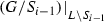, i.e., the result of removing the vertices in $$S_{i-1}$$ and all their incident edges. For $$i = 1, \ldots , k$$, we argue by induction on i that the coverage probabilities of F outside of $$S_{i-1}$$ coincide with those of a maxmin-fair distribution for $$H_i$$; and and moreover the probabilities are as prescribed by the statement of the lemma.

The case $$i = 1$$ is trivial, so assume $$i > 1$$. By Corollary 2, there is a distribution of matchings in $$H_i$$ with minimum satisfaction probability at least $$\lambda _i$$; the expected number of covered elements from $$B_i$$ is then at least $$\lambda _i |B_i| = |\varGamma (B_i) {\setminus } \varGamma (S_{i-1})| = |\varGamma _{H_i}(B_i)|$$. Hence equality must always hold, and the maxmin-fair distribution $$F_i$$ for $$H_i$$ has satisfaction probability precisely $$\lambda _i$$ for all $$u \in B_i$$. By the induction hypothesis, $$F[u] = F_i[u] = \lambda _i$$ for all $$u \in B_i$$. Now observe that the neighbors of $$B_i$$ that belong to $$S_{i-1}$$ are already matched with probability 1. There are only $$|\varGamma _{H_i}(B_i)|$$ other neighbors, and since the expected number of covered neighbours of $$B_i$$ in F is equal to $$|\varGamma _{H_i}(B_i)|$$, it follows that any $$w \in \varGamma _{H_i}(B_i)$$ is matched to some $$v \in B_i$$ with probability 1 in F. In particular, in F no element of $$\varGamma _{H_i}(B_i)$$ is matched to any vertex outside $$B_i$$ with non-zero probability, so the satisfaction probabilities of F outside of $$S_i$$ must coincide with those of of a maxmin-fair distribution for $$H_{i+1}$$. $$\square$$

### Lemma 11

For any two distinct fairly isolated sets X and Y, either $$X \subseteq Y$$ or $$Y \subseteq X$$ holds.

### Proof

By Corollaries 2 and 3, $$X \ne \emptyset$$ is fairly isolated if and only if $$X = L$$ orFor any two sets AB such that $$A \subsetneq B$$, define $$d(A \mid B) = \frac{ |\varGamma (A \cup B)| - |\varGamma (B)| }{|A {\setminus } B|}.$$ Then we can rewrite the definition of fair isolation (including the case $$X = L$$) as:

\begin{aligned} X\text { is fairly isolated} \qquad \Leftrightarrow \qquad d(X \mid S) < d(T \mid X) \qquad \forall \; S\subsetneq X, T\supsetneq X. \end{aligned}

Now assume for contradiction X and Y are fairly isolated but $$X {\setminus } Y$$ and $$Y{\setminus } X$$ are both non-empty. Then $$d(X \mid X \cap Y)$$ and $$d(Y\mid X \cap Y)$$ are well defined; assume without loss of generality that $$d(X \mid X \cap Y) \le d(Y\mid X \cap Y)$$. Then

\begin{aligned} d(X\mid X \cap Y) \le d(Y\mid X \cap Y) < d(X \cup Y\mid Y), \end{aligned}

where we used the fair isolation of Y. But this contradicts the submodularity of $$\varGamma$$. $$\square$$

### Proof of Theorem 5

Let $$B'_1, \ldots , B'_k$$ be the sequence of sets given by Lemma 10 and define $$S'_i = \bigcup _{j \le i} B'_i,$$$$\lambda '_i = \frac{\varGamma (S'_i \cup B'_i) - \varGamma (S'_i) }{|B'_i|}$$ and $$\lambda '_0 = 0$$. We show that $$S'_1, \ldots , S'_{k}$$ comprise all fairly isolated sets. Assuming this for the moment, notice that by definition these sets form a chain, and the sets $$B'_1, \ldots , B'_k$$ satisfy property (a) by Lemma 10. Part (b) follows then by applying the fair decomposition to the dual of the matching matroid, using Corollary 3, and recalling that maxmin-fairness and minmax-Pareto efficiency are equivalent for matroids (Theorem 2).

To see that $$S'_1, \ldots , S'_{k-1}$$ are fairly isolated, notice that $$S'_k = L$$ indeed is by definition, whereas for $$i < k$$ we have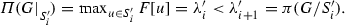This meets the definition of fair separation from Sect. 4.4.

The fact that the fairly isolated sets form a chain is a direct consequence of Lemma 11. Finally, assume for contradiction that some fairly isolated set X exists other than $$S'_1, \ldots , S'_k$$. Then $$S'_i \subsetneq X \subsetneq S'_{i+1}$$ for some $$i, 0 \le i < k$$. Then we have

\begin{aligned} \lambda '_{i+1} \le \frac{ |\varGamma (X)| - |\varGamma (S'_i)| }{|X{\setminus } S'_i|} < \frac{ |\varGamma (S'_{i+1})| - |\varGamma (X)| }{|S'_{i+1}{\setminus } X|}, \end{aligned}
(22)

where the first inequality is by construction of $$B'_{i+1}$$ and $$S'_{i+1}$$, and the second by the fair isolation of X. But then

\begin{aligned} \lambda '_{i+1} {|S'_{i+1}{\setminus } X|} + |\varGamma (X)| < |\varGamma (S'_{i+1})| =|\varGamma (S'_{i})| + \lambda '_{i+1} |B'_i|, \end{aligned}

i.e.,

\begin{aligned} |\varGamma (X)| - |\varGamma (S'_{i})|< \lambda '_{i+1} {|X{\setminus } S'_{i}|}, \end{aligned}